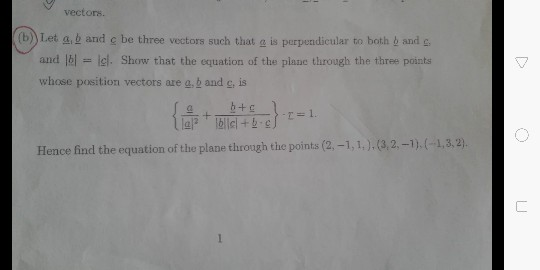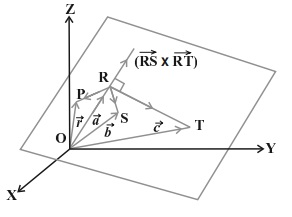# vectors (b)) Let a, b and c be three vectors such that a is perpendicular to both b and and Ibl = lel. Showr that th...vectors (b)) Let a, b and c be three vectors such that a is perpendicular to both b and and Ibl = lel. Showr that the equation of the plane through the three points whose position vectors are g, b and c, is le尸. }blicl+b.ef Hence find the equation of the plane through the points (2,-1,1,).(3,2,-1).(-1,3.2) ㄈ

As the name suggests, non collinear points refer to those points that do not all lie on the same line. From our knowledge from previous lessons, we know that an infinite number of planes can pass through a given vector that is perpendicular to it but there will always be one and only one plane that is perpendicular to the vector and passes through a given point.

In this lesson, we shall focus on the equation of a plane passing through three non collinear points. We shall look at both – the Vector form and the Cartesian form of equations.

Vector Form

Let us consider three non collinear points – R, S, T. Let the position vectors of these points be a⃗ ,bandc⃗ . We know that the product of the vectors RSandRT→ must be perpendicular to the plane containing the points R, S and T.Source: examfear

Let us also assume that the position vector of the point P is r⃗ and that of point R is a⃗ . Again, we know that the equation of the plane perpendicular to RS→×RT→ and passing through point P must be

(r⃗ –a⃗ ).(RS→×RT→)=0

From the figure we can write,

(r⃗ –a⃗ ).[(b⃗ –a⃗ )×(c⃗ –a⃗ )]=0

Note that here b⃗ and c⃗ are the position vectors of the points S and T respectively. Thus, the above equation can be taken to represent the equation of a plane passing through three non collinear points in Vector form.

We shall now move on to the Cartesian equation.

Cartesian Form

Let us now consider the coordinates of the three non collinear points as under –

(R(x1,y1,z1),S(x2,y2,z2),T(x3,y3,z3)

Let P be a point on the plane that must contain these points. The position vector of the point P be r⃗ and its coordinates are (x, y, z). Now, we can write the vectors as –

RP→=(xx1)i^+(yy1)j^+(zz1)k^

RS→=(x2–x1)i^+(y2–y1)j^+(z2–z1)k^

Also,

RP→=(x3–x1)i^+(y3–y1)j^+(z3–z1)k^

We can now use the Vector form to substitute the above vectors. Doing so would give us the advantage of presenting the equation in determinant form as under –

∣∣∣∣∣(xx1)(x2–x1)(x3–x1)(yy1)(y2–y1)(y3–y1)(zz1)(z2–z1)(z3–z1)∣∣∣∣∣=0

Thus the determinant form gives us nothing but the Cartesian equation of a plane passing through three non collinear points.

Solved Example for You

Question: Find the equation of the plane in Vector form that passes through the points (1, 1, 0), (1, 2, 1) and (-2, 2, -1).

Answer: We shall first check the determinant of the three points to check for collinearity of the points.

⎡⎣⎢11−212201−1⎤⎦⎥=−8

Since the value of the determinant is not zero, it implies that the points are non collinear. Now, we shall find the equation of the plane in Cartesian from as under –

∣∣∣∣∣(x–1)(1–1)(−2–1)(y–1)(2–1)(2–1)(z–0)(1–0)(−1–0)∣∣∣∣∣=0

∣∣∣∣(x–1)0−3(y–1)11z1−1∣∣∣∣=0

On solving, we shall get :

(x – 1) (-2) – (y – 1) (-3) + z (-3) = 0

-2x + 3y -3z -1 = 0

2x – 3y + 3z = -1 is the required equation of the plane.

##### Add Answer of: vectors (b)) Let a, b and c be three vectors such that a is perpendicular to both b and and Ibl = lel. Showr that th...
More Homework Help Questions Additional questions in this topic.